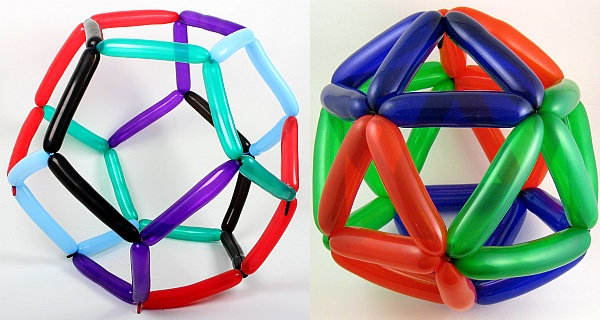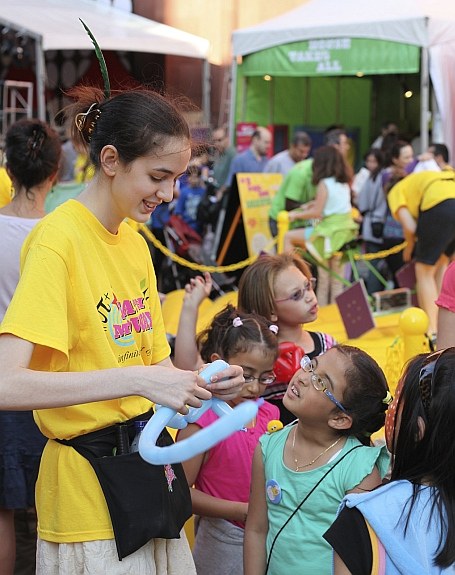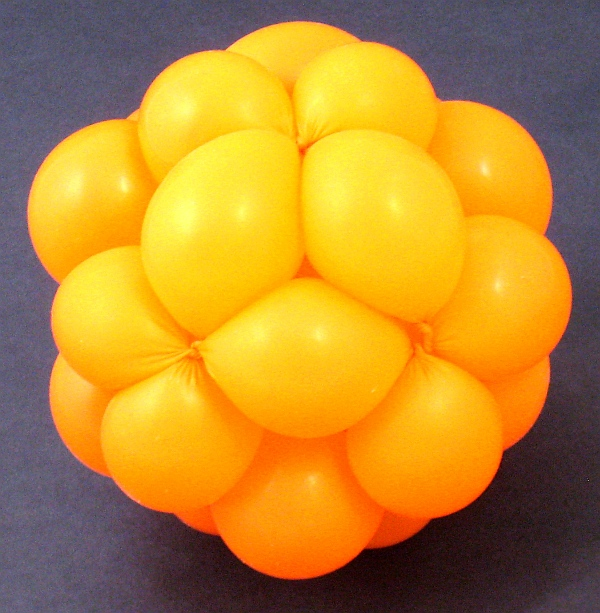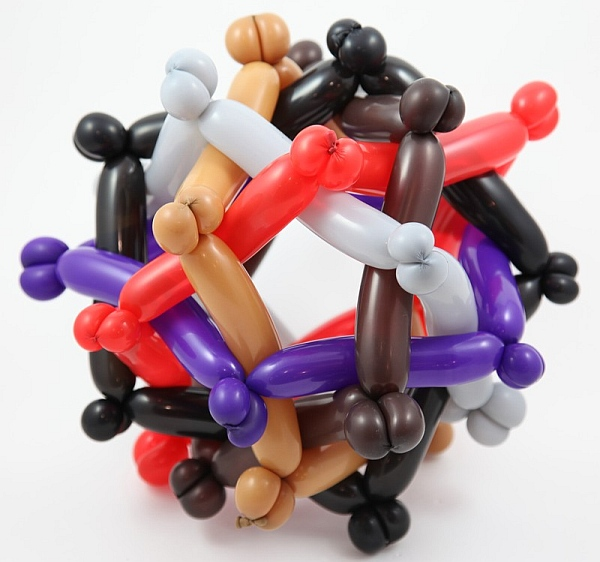Math Monday: Polyhedral Balloons
MARCH 1, 2010

by George HartFor making mathematical models of polyhedra, a convenient and inexpensive material is the long clown ballon.  This dodecahedron (made of ten balloons) and icosahedron (made of six balloons) are two examples from a study of Mathematical Balloon Twisting by Erik Demaine, Marty Demaine, and Vi Hart.At several Math Midway events, the Museum of Mathematics has been pleased to have a balloon polyhedron expert twist balloons into octahedra for the museum visitors. Here, Vi Hart is making an octahedron from one balloon.With practice, one can work up to more complex models, such as this cuboctahedron made from a single balloon. The balloon outlines the twenty four edges of a cuboctahedron, which consists of eight triangles and six squares.Even more spectacular is this “orderly tangle” of six concentric regular pentagons, made from six balloons. Detailed instructions to start you making mathematical balloon constructions are available here.